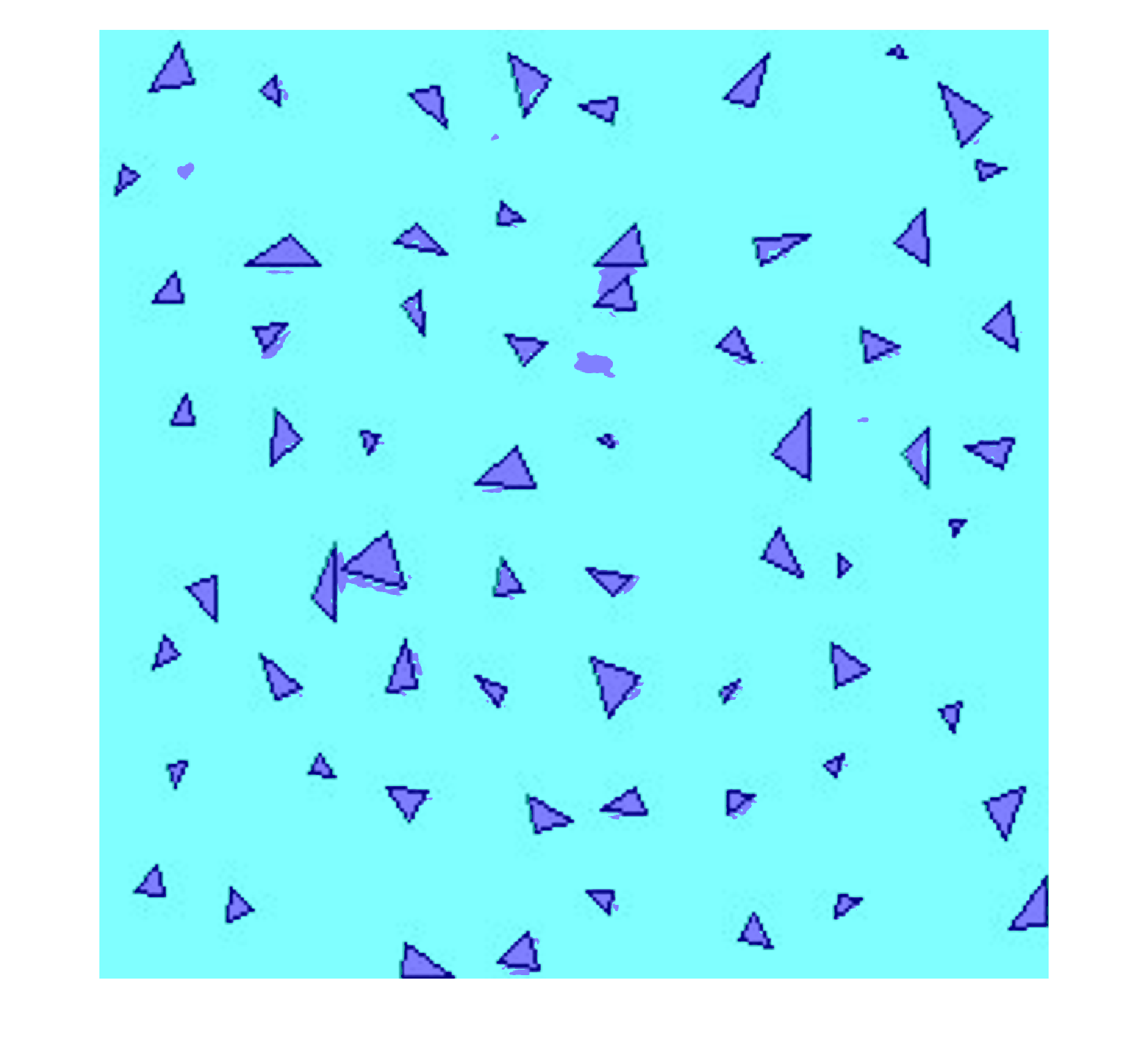# deeplabv3plusLayers

Create DeepLab v3+ convolutional neural network for semantic image segmentation

## Syntax

``layerGraph = deeplabv3plusLayers(imageSize,numClasses,network)``
``layerGraph = deeplabv3plusLayers(___,'DownsamplingFactor',value)``

## Description

example

````layerGraph = deeplabv3plusLayers(imageSize,numClasses,network)` returns a DeepLab v3+ layer with the specified base network, number of classes, and image size.```
````layerGraph = deeplabv3plusLayers(___,'DownsamplingFactor',value)` additionally sets the downsampling factor (output stride)  to either `8` or `16`. The downsampling factor sets the amount the encoder section of DeepLav v3+ downsamples the input image.```

## Examples

collapse all

Create a DeepLab v3+ network based on ResNet-18.

```imageSize = [480 640 3]; numClasses = 5; network = 'resnet18'; lgraph = deeplabv3plusLayers(imageSize,numClasses,network, ... 'DownsamplingFactor',16);```

Display the network.

`analyzeNetwork(lgraph)`

Load the triangle data set images using an image datastore. The datastore contains 200 grayscale images of random triangles. Each image is 32-by-32.

```dataSetDir = fullfile(toolboxdir('vision'),'visiondata','triangleImages'); imageDir = fullfile(dataSetDir,'trainingImages'); imds = imageDatastore(imageDir);```

Load the triangle data set pixel labels using a pixel label datastore.

```labelDir = fullfile(dataSetDir, 'trainingLabels'); classNames = ["triangle","background"]; labelIDs = [255 0]; pxds = pixelLabelDatastore(labelDir,classNames,labelIDs);```

Create a DeepLab v3+ network.

```imageSize = [256 256]; numClasses = numel(classNames); lgraph = deeplabv3plusLayers(imageSize,numClasses,'resnet18');```

Combine image and pixel label data for training and apply a preprocessing transform to resize the training images.

```cds = combine(imds,pxds); tds = transform(cds, @(data)preprocessTrainingData(data,imageSize));```

Specify training options. Lower the mini-batch size to reduce memory usage.

```opts = trainingOptions('sgdm',... 'MiniBatchSize',8,... 'MaxEpochs',3);```

Train the network.

`net = trainNetwork(tds,lgraph,opts);`
```Training on single GPU. Initializing input data normalization. |========================================================================================| | Epoch | Iteration | Time Elapsed | Mini-batch | Mini-batch | Base Learning | | | | (hh:mm:ss) | Accuracy | Loss | Rate | |========================================================================================| | 1 | 1 | 00:00:00 | 34.79% | 0.9817 | 0.0100 | | 2 | 50 | 00:00:23 | 99.02% | 0.0261 | 0.0100 | | 3 | 75 | 00:00:31 | 99.16% | 0.0211 | 0.0100 | |========================================================================================| ```

`I = imread('triangleTest.jpg');`

Resize the test image by a factor equal to the input image size divided by 32 so that the triangles in the test image are roughly equal to the size of the triangles during training.

`I = imresize(I,'Scale',imageSize./32);`

Segment the image.

`C = semanticseg(I,net);`

Display the results.

```B = labeloverlay(I,C); figure imshow(B)```Supporting Functions

```function data = preprocessTrainingData(data, imageSize) % Resize the training image and associated pixel label image. data{1} = imresize(data{1},imageSize); data{2} = imresize(data{2},imageSize); % Convert grayscale input image into RGB for use with ResNet-18, which % requires RGB image input. data{1} = repmat(data{1},1,1,3); end```

## Input Arguments

collapse all

Network input image size, specified as a:

• 2-element vector in the format [height, width].

• 3-element vector in the format [height, width, `3`]. The third element, 3, corresponds to RGB.

Number of classes for network to classify, specified as an integer greater than 1.

Base network, specified as `resnet18` (Deep Learning Toolbox), `resnet50` (Deep Learning Toolbox), `mobilenetv2` (Deep Learning Toolbox), `xception` (Deep Learning Toolbox), or `inceptionresnetv2` (Deep Learning Toolbox). You must install the corresponding network add-on.

## Output Arguments

collapse all

DeepLab v3+ network, returned as a convolutional neural network for semantic image segmentation. The network uses encoder-decoder architecture, dilated convolutions, and skip connections to segment images. You must use the `trainNetwork` (Deep Learning Toolbox) function (requires Deep Learning Toolbox™) to train the network before you can use the network for semantic segmentation.

## Algorithms

• When you use either the `xception` (Deep Learning Toolbox) or `mobilenetv2` (Deep Learning Toolbox) base networks to create a DeepLab v3+ network, depth separable convolutions are used in the atrous spatial pyramid pooling (ASPP) and decoder subnetworks. For all other base networks, convolution layers are used.

• This implementation of DeepLab v3+ does not include a global average pooling layer in the ASPP.

 Chen, L., Y. Zhu, G. Papandreou, F. Schroff, and H. Adam. "Encoder-Decoder with Atrous Separable Convolution for Semantic Image Segmentation." Computer Vision — ECCV 2018, 833-851. Munic, Germany: ECCV, 2018.